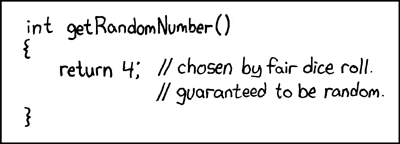# What is an example of complex random string, in the Kolmogorov-Chatin sense?

Any string generated from a PRNG clearly has a very short description. You need the code for the random number generator, the seed, and then the number of times to iterate. So, it seems that all such strings have low KC complexity.

If the above it true, then what is an example of a "complex" string, in the Kolmogorov-Chatin sense?

• You can pick a bunch of bits from random.org
– Vor
Feb 11, 2013 at 9:15
• A͇̠̭͕̙̻̍̈́͗ͩ͠ ̉̔ͤ̓͌̉̽͠"͍͉̰͎ͨ̓̃c͕̟̈́ͧ̒̽̚͟o̰͈̠ͭm͔̭̳͙͉̪͌̅̔ͦp̷̺̱̠ͪ͂͌ͨ͂̚l̡̓̄̐ê̚͞x̖̟̝̻̫̻̙ͧͩ̎̆"̜͈ͪͤͥ͡ ̩̫͓ͭͯ̀s̥̼͂̃̿ͮ͊ṭ̫̺̬ͯͤ͛r̗̯͊ͣ̄̓ͪi͕̟̲̝̖͎ͨ̋̓̉̈́ͦn̹̟̗͚͒̋̏͂̂g̜ͫ̄̐̔͒ͤ,͈̫̟̬͊̔̑̐͐ ̪̝̟̼̜̩͒ͩ̈͌̓ȋ̹̟̺̪͉̺ͩͥ͋̅͂̏̕n̜͆ ̯̭t̖ͩ͛h̺͓͚̹ͯ̒̽ͮͫ͠e̪̹̓̇͌̒̎ͥ̋ ̫ͤ́ͦ͂̒͗̃͢K̍҉̘͔̭̪̰̦̺o̱͚̰͙̺̥̒͆͒̀l̺͉̬̤̬̊̕m̨͖̦̻̥ͮͦ̏͊͆o͈͒͂ͩ͆g̭͙̼̦o̞̤̮̲͑ͩ͞r̺͕̩͓̜̯͇̊ͭ͂̍̇̋̓o̙͉͍̭̟͖v̦̏ͭ̀̔̅̈ͧ-͗̑҉͉C͚̱̣͎͚͔͆̕h̖̽͗ͧͨͤ͘a̞͐͌͆̅̍̾ṭ̻̗̺̫̖̭ͬ͑̍ͤͪ͊̂i̼͎̻̹̲̟͎ͬ̾̄̉̐ͥn̗̐ͧ̀ ̔͒̇ͤͪ͊̎͡s̶̳̖̥̥̊ͪͦ͑̐̐e̝̖͓̺̤̰̗̍ͯ̓̈́̋͡n҉̻̮s͉͈̣̫͍͂̕e͔̜͓ͬ̒̂̐ͅ Feb 11, 2013 at 17:10
• I think what you want to ask is not just an example, you are probably confusing Kolmogorov random strings (i.e. strings with high Kolmogorov complexity) with pseudorandomness. If that is the case please clarify the question and ask for example "is there a relation between strings with high Kolmogorov complexity and pseudorandomness?". Feb 12, 2013 at 23:37

It is impossible to give a specific example of a string with high Kolmogorov complexity. If I was to give an example in this answer, then the following piece of code would retrieve it:

wget http://cs.stackexchange.com/q/9721


(plus some O(1) post-processing).

A string of high Kolmogorov complexity is as elusive as a random number:There is in fact a connection between randomness and Kolmogorov complexity: a random string has maximal complexity for its length. (At least for one definition of randomness, appropriately called Kolmogorov randomness.)

This observation that it is impossible to exhibit a string of high Kolmogorov complexity can be put into a mathematical form and proved: it is called Chaitin's theorem.

The high-complexity strings are all the ones that you cannot describe.

• Your example with "wget ..." is nice, it let me think about it, but I guess it is not appropriate. Kolmogoroff complexity is defined relative to some universal computation mechanism. So consider the internet and all computers connected to it as such a mechanism and think it fixed, then a random number is some number that is not written anywhere on the web and could not be described by any programming language on any server/computer on the web shorter than itself. Of course there are such strings, I can write it down on paper, but when I write it here then I alter the web, i.e. it is like... Jul 25, 2015 at 0:02
• ... reprogramming your universal computing mechanism, i.e. hardwiring the string into your computing mechanism, then of course w.r.t. to this new mechanism it is no longer random. This is similar to the formal statement that for every string $x$ there exists some universal Turing machine $U$ such that $C_U(x) = 0$ (i.e. the Kolm. complexity w.r.t. $U$), just hardwire the number into $U$ and print it for some simple input or by default. But anyway you made a good point in stipulating to think about this issue, would made up a good exercise ;) Jul 25, 2015 at 0:05

New research since this question was asked may actually provide an answer, if I read it correctly.

One of the most complex* 12 bit strings in the Kolmogorov sense is 001111000011, relative to all 5 state, 2 symbol Turing machines. It has a Kolmogorov complexity of approximately 37.06… bits, which is 11.45… bits higher than the simplest 12 bit strings. (which are twelve 0 bits in a row or 1 bits in a row)

* The inverse bit string has the same complexity. There are also two 12 bit strings that are uncomputable by 5 state Turing machines, so likely have a slightly higher Kolmogorov complexity.

The infinite string that has a 1 at the $i$-th position if the $i$-th Turing machine (in some fixed enumeration of TMs) halts on all inputs has a very high complexity.

Here is one example of a random string in the Kolmogorov sense:

kejrjtokplarkoprl[paxes,0owp,itro,se0ofiespoKWP[OLAOINJ-O2CLKSEJOPOS[P3VTOIIOIopqwiodhriuropdiorpdioieurfpoiopdioiuoidurodpiwoptdopirtois02-9980uhoititoci0ieioq34u0-40brnjvhw09a3=-rjchgo0ewir

• In my programming language, that string is exactly the result of a builtin function PrintGarbage(). Feb 11, 2013 at 13:46
• See my comment on "Gilles" post, this applies here too, by the PrintGarbage function you alter your computation mechanism. The programming languages I know do not have such a function, so with respect to the programming languages I know the string above might be truly random, despite not with respect to your programming language as description mechanism. Jul 25, 2015 at 0:10
• Not likely... the above string is 191 bytes uncompressed, 187 bytes gzip compressed. May 10, 2017 at 18:50